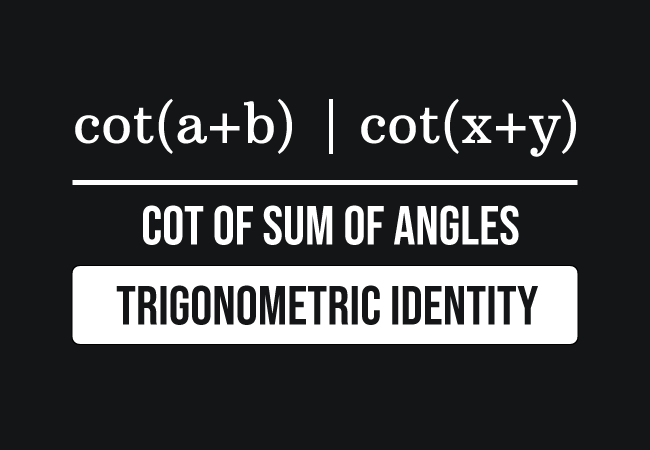# Cot of angle sum identity

## Formula

$(1).\,\,$ $\cot{(a+b)}$ $\,=\,$ $\dfrac{\cot{b}\cot{a}-1}{\cot{b}+\cot{a}}$

$(2).\,\,$ $\cot{(x+y)}$ $\,=\,$ $\dfrac{\cot{y}\cot{x}-1}{\cot{y}+\cot{x}}$

### Introduction

Let’s assume that $a$ and $b$ are two variables, which are used to represent two angles. In mathematics, the sum of two angles is written as $a+b$, which is actually a compound angle. The cotangent of a compound angle $a$ plus $b$ is written as $\cot{(a+b)}$ in trigonometric mathematics.The cotangent of the sum of angles $a$ and $b$ is equal to the quotient of the subtraction of one from the product of cotangents of angles $a$ and $b$ by the sum of the cotangents of angles $a$ and $b$.

$\cot{(a+b)}$ $\,=\,$ $\dfrac{\cot{b} \times \cot{a}-1}{\cot{b}+\cot{a}}$

This mathematical equation is called the cotangent of angle sum trigonometric identity in mathematics.

#### Usage

The cot angle sum identity is possibly used in two cases in trigonometric mathematics.

##### Expansion

The cot of the sum of two angles is expanded as the quotient of the subtraction of one from the product of cotangents of angles by the sum of the cotangents of angles.

$\implies$ $\cot{(a+b)}$ $\,=\,$ $\dfrac{\cot{(b)}\cot{(a)}-1}{\cot{(b)}+\cot{(a)}}$

##### Simplification

The quotient of the subtraction of one from the product of cotangents of angles by the sum of the cotangents of angles is simplified as the cot of the sum of two angles.

$\implies$ $\dfrac{\cot{(b)}\cot{(a)}-1}{\cot{(b)}+\cot{(a)}}$ $\,=\,$ $\cot{(a+b)}$

#### Forms

The angle sum trigonometric formula in cotangent function is written in several forms but it is popularly expressed in the following three forms.

$(1).\,\,$ $\cot{(A+B)}$ $\,=\,$ $\dfrac{\cot{B}\cot{A}-1}{\cot{B}+\cot{A}}$

$(2).\,\,$ $\cot{(x+y)}$ $\,=\,$ $\dfrac{\cot{y}\cot{x}-1}{\cot{y}+\cot{x}}$

$(3).\,\,$ $\cot{(\alpha+\beta)}$ $\,=\,$ $\dfrac{\cot{\beta}\cot{\alpha}-1}{\cot{\beta}+\cot{\alpha}}$

#### Proof

Learn how to derive the cot of angle sum trigonometric identity by a geometric method in trigonometry.

A best free mathematics education website that helps students, teachers and researchers.

###### Maths Topics

Learn each topic of the mathematics easily with understandable proofs and visual animation graphics.

###### Maths Problems

A math help place with list of solved problems with answers and worksheets on every concept for your practice.

Learn solutions

###### Subscribe us

You can get the latest updates from us by following to our official page of Math Doubts in one of your favourite social media sites.